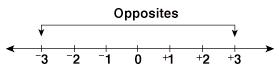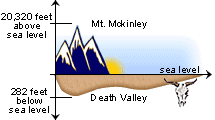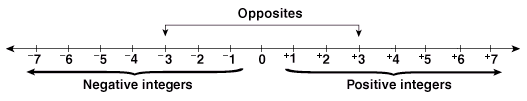# What is an Integer?

## Integers Lesson and Integer Examples

Use the following examples and interactive exercises to learn about Integers, also can be called numbers.Problem: The highest elevation in North America is Mt. McKinley, which is 20,320 feet above sea level. The lowest elevation is Death Valley, which is 282 feet below sea level. What is the distance from the top of Mt. McKinley to the bottom of Death Valley?

Solution: The distance from the top of Mt. McKinley to sea level is 20,320 feet and the distance from sea level to the bottom of Death Valley is 282 feet. The total distance is the sum of 20,320 and 282, which is 20,602 feet.

The problem above uses the notion of opposites: Above sea level is the opposite of below sea level. Here are some more examples of opposites:

top, bottom  |  increase, decrease  |  forward, backward  |  positive, negative

We could solve the problem above using Integers, the set of whole numbers and their opposites. The number line below is used to represent them.### Definitions

• The number line goes on forever in both directions. This is indicated by the arrows.
• Whole numbers greater than zero are called positive integers (+). These numbers are to the right of zero on the number line.
• Whole numbers less than zero are called negative integers (-). These numbers are to the left of zero on the number line.
• Zero is neutral. It is neither positive nor negative.
• Two numbers are opposites if they are each the same distance away from zero, but on opposite sides of the number line. One will have a positive sign, the other a negative sign. In the number line above, +3 and -3 are labeled as opposites.Problem: The highest elevation in North America is Mt. McKinley, which is 20,320 feet above sea level. The lowest elevation is Death Valley, which is 282 feet below sea level. What is the distance from the top of Mt. McKinley to the bottom of Death Valley?

Solution: We can represent the elevation using positive and negative numbers:

 Elevation Integer 20,320 feet above sea level +20,320 sea level 0 282 feet below sea level -282

The distance from the top of Mt. McKinley to the bottom of Death Valley is the same as the distance from +20,320 to -282 on the number line. We add the distance from +20,320 to 0, and the distance from 0 to -282, for a total of 20,602 feet.

Example 1: Write a number using positive or negative signs numberto represent each situation:

 10 degrees above zero+10 a loss of 16 dollars-16 a gain of 5 points+5 8 steps backward-8Example 2: Name the opposite of each integer.

 -12+12 +21-21 -17+17 +9-9Example 3: Name 4 real life situations in which integers can be used.

Spending and earning money.

Rising and falling temperatures.

Stock market gains and losses.

Gaining and losing yards in a football game.Note: A positive number does not have to have a + sign in it. For example, +3 and 3 are interchangeable.

Summary: Integers are the set of whole numbers and their opposites. Whole numbers greater than zero are called positive. Whole numbers less than zero are called negative. Zero is neither positive nor negative, and has no sign. Two integers are opposites if they are each the same distance away from zero, but on opposite sides of the number line.### Exercises

Directions: Read each question below. Click once in an ANSWER BOX and type in your answer; then click ENTER. Do not enter commas in your answers. After you click ENTER, a message will appear in the RESULTS BOX to indicate whether your answer is correct or incorrect. To start over, click CLEAR. Use the + key to write a positive number, and the - key to write a negative number. Omit words and labels from your answers.

 1.Write an integer to represent the following situation: Earnings of 15 dollars ANSWER BOX:   RESULTS BOX:
 2.Write an integer to represent the following situation: A loss of 20 yards ANSWER BOX:   RESULTS BOX:
 3.What is the opposite of -231? ANSWER BOX:   RESULTS BOX:
 4.What is the opposite of +1096? ANSWER BOX:   RESULTS BOX:
 5.Solve the following problem using integers. Jenny has \$2. She earns \$5, spends \$10, earns \$4, then spends \$3. How many dollars does she have or owe? ANSWER BOX: \$  RESULTS BOX: